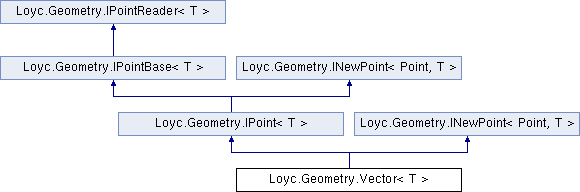Enhanced C# Language of your choice: library documentation

# Documentation moved to ecsharp.net

GitHub doesn't support HTTP redirects, so you'll be redirected in 3 seconds.

Loyc.Geometry.Vector< T > Struct Template Reference

Represents a two-dimensional vector, i.e. a magnitude and direction or the difference between two points, stored as X and Y components. More...

Source file:
Inheritance diagram for Loyc.Geometry.Vector< T >:## Remarks

Represents a two-dimensional vector, i.e. a magnitude and direction or the difference between two points, stored as X and Y components.

A vector is the same as a point except for the operations it supports. For example, it usually does not make sense to add two points, but you can add two vectors (to get another vector) or you can add a vector to a point (to get a point).

If you really do need to add two points together or something like that, you can typecast from Point{T} to Vector{T}.

Although this structure contains operators such as + and -, their performance is suboptimal due to limitations of C#. The PointMath class of Loyc.Utilities.dll contains extension methods such as Add() and Sub() that perform faster on common coorindate types such as int and double.

Loyc.Geometry.PointMath, Loyc.Geometry.VectorMath{T}, Point{T}
Type Constraints
 T : IConvertible T : IEquatable

_y

## Public static fields

static ISignedMath< T > m = Maths<T>.SignedMath

static readonly Vector< T > Zero = new Vector<T>()

static readonly Vector< T > Inf = new Vector<T>(m.PositiveInfinity, m.PositiveInfinity)

## Properties

X` [get, set]`

Y` [get, set]`Properties inherited from Loyc.Geometry.IPointBase< T >
new T X` [get, set]`
Horizontal coordinate of a point or vector. More...

new T Y` [get, set]`
Vertical coordinate of a point or vector. More...Properties inherited from Loyc.Geometry.IPointReader< T >
X` [get]`

Y` [get]`

## Public Member Functions

Vector (T x, T y)

override bool Equals (object other)

override int GetHashCode ()

override string ToString ()

Vector< T > New (T x, T y)

IPoint< T > INewPoint< IPoint
< T >, T >.
New (T x, T y)

bool Equals (Vector< T > other)

## Static Public Member Functions

static operator Point< T > (Vector< T > p)

static operator Vector< int > (Vector< T > p)

static operator Vector< long > (Vector< T > p)

static operator Vector< float > (Vector< T > p)

static operator Vector< double > (Vector< T > p)

static Vector< T > operator+ (Vector< T > a, Vector< T > b)

static Vector< T > operator- (Vector< T > a, Vector< T > b)

static Vector< T > operator* (Vector< T > p, T factor)

static Vector< T > operator/ (Vector< T > p, T factor)

static Vector< T > operator<< (Vector< T > p, int amt)

static Vector< T > operator>> (Vector< T > p, int amt)

static T operator* (Vector< T > a, Vector< T > b)
Dot product. a*b equals lhs.Length*rhs.Length*Cos(theta) if theta is the angle between two vectors. More...

static Vector< T > operator- (Vector< T > a)
Reverses a vector. More...

static bool operator== (Vector< T > a, Vector< T > b)

static bool operator!= (Vector< T > a, Vector< T > b)

## Member Function Documentation

 static T Loyc.Geometry.Vector< T >.operator* ( Vector< T > a, Vector< T > b )
inlinestatic

Dot product. a*b equals lhs.Length*rhs.Length*Cos(theta) if theta is the angle between two vectors.

 static Vector Loyc.Geometry.Vector< T >.operator- ( Vector< T > a )
inlinestatic

Reverses a vector.